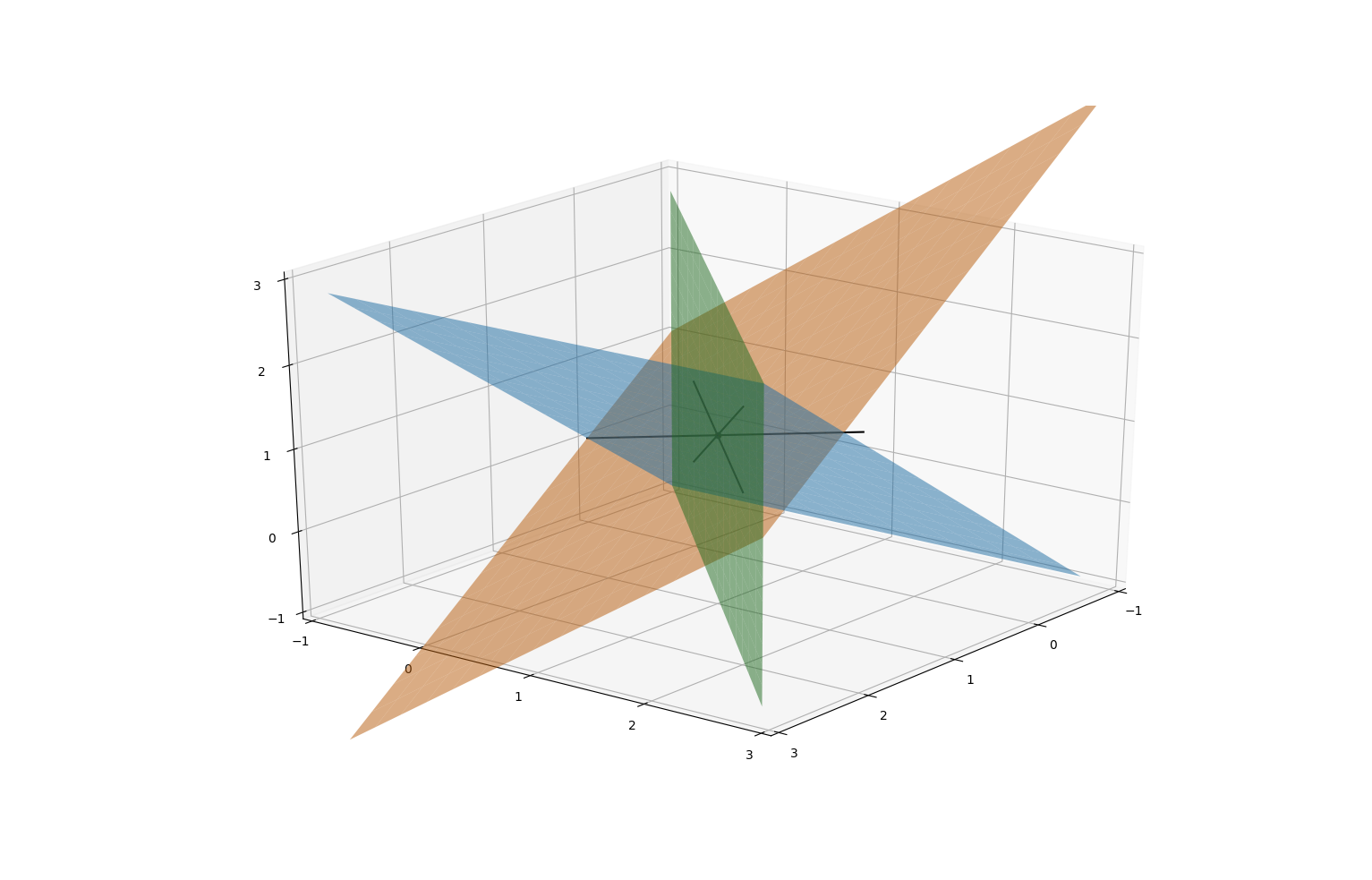# 18. Geometric viewpoint on simultaneous equations, 2

## 18. Geometric viewpoint on simultaneous equations, 2

### 3 variables

Now let's look at higher dimensional systems.

Consider the equations $x-y+z=1,\qquad x-z=0.$ The set of points whose coordinates satisfy these equations is a subset of 3-dimensional space $\mathbf{R}^{3}$ . Each equation cuts out a plane; their intersection (the set of simultaneous solutions) is a line. :The solution of the equations is $z=x$ , $y=2x-1$ ($x$ is a free variable); this gives us a parametrisation of the line of intersection: it consists of vectors of the form $\begin{pmatrix}x\\ 2x-1\\ x\end{pmatrix}$ .

Each equation $a_{1}x+a_{2}y+a_{3}z=b$ gives us a plane. This plane is orthogonal to the vector $\begin{pmatrix}a_{1}\\ a_{2}\\ a_{3}\end{pmatrix}$ . The $z$ -intercept is where $x=y=0$ , so $z=b/a_{3}$ .

Let's consider the system $x-y+z=1,\qquad x-z=0,\qquad x-y=0.$ The first two planes are the same as before; the third equation gives a third plane. The set of solutions is the set of triple intersections between these planes. Solving, we get $x=y=z$ and $z=y=2z-1$ , so $x=y=z=1$ . Therefore there is a unique triple intersection point at $(1,1,1)$ .### Higher dimensions

An equation $a_{1}x_{1}+\cdots+a_{n}x_{n}=b$ in $n$ variables defines a hyperplane in $n$ -dimensional space $\mathbf{R}^{n}$ . This is the hyperplane orthogonal to the vector $\begin{pmatrix}a_{1}\\ \cdots\\ a_{n}\end{pmatrix}$ .

If $v=\begin{pmatrix}x_{1}\\ \vdots\\ x_{n}\end{pmatrix}$ is a vector of variables and $Av=b$ is a system of $m$ simultaneous equations encoded with an $m$ -by-$n$ matrix $A$ then each row of $A$ defines a hyperplane $A_{i1}x_{1}+\cdots+A_{in}x_{n}=b_{i}$ in $\mathbf{R}^{n}$ . The set of solutions is a subspace of $\mathbf{R}^{n}$ . Subspace is a catch-all name, which includes points, lines, planes, hyperplanes, and everything in between (for which we don't have everyday words).

Consider the equations $w+x+y+z=0$ and $x-y=1$ . This is a pair of equations in four variables, so defines a subspace of solutions in $\mathbf{R}^{4}$ . Each equation gives a 3-dimensional hyperplane in $\mathbf{R}^{4}$ . Their intersection is a 2-dimensional subspace (plane) living in $\mathbf{R}^{4}$ . Solving the equations: $w=-2y-z$ , $x=1-y$ . We see that $y,z$ are free variables and $w,x$ are dependent variables. The fact that there are two free variables is another way of saying that the subspace of solutions is 2-dimensional: each free variable is a coordinate on the subspace of solutions.

Remark:

More generally, the dimension of the subspace of solutions equals the number of free variables: we can think of the free variables as parameters for describing points in the subspace of solutions.